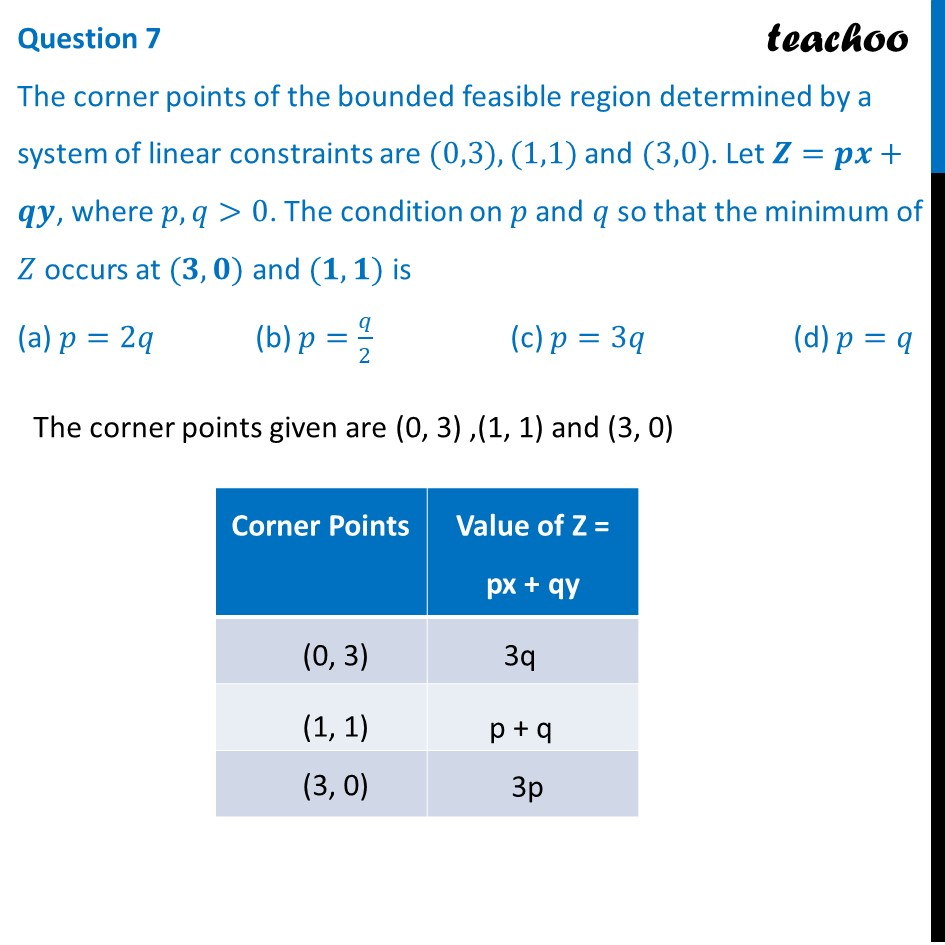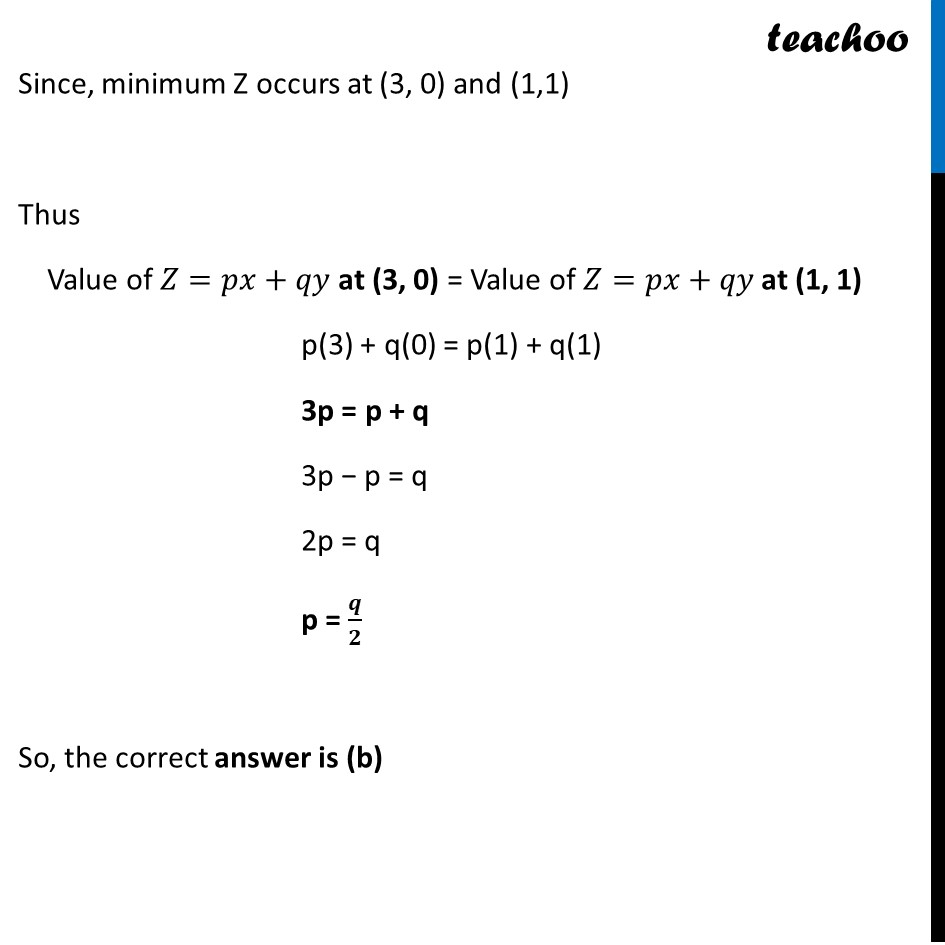CBSE Class 12 Sample Paper for 2024 Boards

Class 12
Solutions of Sample Papers and Past Year Papers - for Class 12 Boards

## (a) p=2q              (b) p=q/2                   (c) p=3q                     (d) p=q

This question is similar to Question 38 Choice-2 CBSE Class 12 Sample Paper for 2021 BoardsLearn in your speed, with individual attention - Teachoo Maths 1-on-1 Class

### Transcript

The corner points given are (0, 3) ,(1, 1) and (3, 0) Since, minimum Z occurs at (3, 0) and (1,1) Thus Value of 𝑍=𝑝𝑥+𝑞𝑦 at (3, 0) = Value of 𝑍=𝑝𝑥+𝑞𝑦 at (1, 1) p(3) + q(0) = p(1) + q(1) 3p = p + q 3p − p = q 2p = q p = 𝒒/𝟐 So, the correct answer is (b)